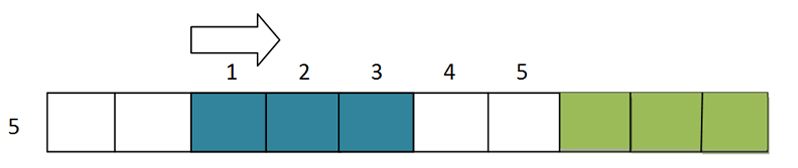How to solve pic-a-pix puzzles:

Blank pic-a-pix grid, now what?

The object of this Japanese puzzle is to form an image by gradually filling in the right squares, based on the number clues.  Begin by choosing a colored pencil crayon, erasable ones are best.  Starting with a blank grid and numbers at the top of columns and left end of rows, you start filling in squares directly adjacent to each other, generally the biggest numbers first.  You should never guess.   You might only fill in one or two squares at a time.  There is no exact order to completing a puzzle.

The 3 basic rules are:

1) The numbers represent how many squares to color in to form a group or block.
2) Leave at least one square between these blocks.
3) The order of the numbers is the order of the blocks, i.e. top number for a column is the top block, and left-most number for a row is the left-most block.

The “Logic Count Method” shown below uses spatial reasoning to enable you to fill in some of the squares and is very useful when you don’t have a clue that reaches the whole length of a row:The number clue shown on left of row is 6, so count 6 squares from the left to right and then count 6 squares from right to left. Confidently shade in overlapping squares.   Even one shaded square can lead to others.   This same method can be applied when there is more than one number, remembering to allow one empty square in-between groups or blocks.

When a group is complete (i.e. all 6 squares are shaded in), and no other clues remain for that row,  mark the remaining squares in that row with a slash (/), or fill them in with a 2nd color which makes for a nice background.   This elimination via shading of background squares is actually very important.

Another case of how elimination of background squares helps you solve the puzzle is shown below.  The clue is 4 and two of the squares are already colored in from vertical clues; count two more squares on each end of the two, which will allow for every possibility of where those last two squares to make up 4 could possibly go.  Using your choice of slashes (/) or a 2nd color, shade in the remaining squares to indicate that they are now background to your image.Another helpful idea is shown below.  The two blue squares are shaded in from vertical clues.  The clue is 5 for this row, so if you count from the extreme left, you can shade in any square that reaches beyond the existing two.And then you can eliminate some squares at the far right, as it is obvious that 5 squares can only extend as far as two more squares over.